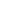Making Thumbnails (Easy)This example shows how to enumerate through a set of files creating thumbnail images from the originals.
 1Creating the Objects We start by creating the variables and a list of files given a file folder. [VBScript] ```Set fso = CreateObject("Scripting.FileSystemObject") Set fldr = fso.GetFolder(Server.MapPath("Picture Folder")) Set canvas = CreateObject("ImageGlue7.Canvas")```
 2Enumerating through the folder and creating thumbnails. Now we can enumerate through the list of files, creating thumbnails of each file.  We do this by telling ImageGlue that we want the image height to be less than or equal to 120 pixels.  If the original height of the image is less than 120 pixels, the original size of the image will be used, otherwise the width will be calculated based on a height of 120 pixels and the aspect ratio of the original image. Also note that we could substitute the 'height<=120' parameter setting with 'width<=120' to limit the width instead of the height. [VBScript] ```For Each theFile In fldr.Files   theFile = theFile.Path   theName = theFile.Name   canvas.DrawFile(theFile, "height<=120")   canvas.SaveAs(Server.MapPath("Thumbnail Folder") & theName & ".jpg", "")   canvas.Clear Next```
 7Input and Output Sample output images are shown below.original.jpgthumbnail.jpg